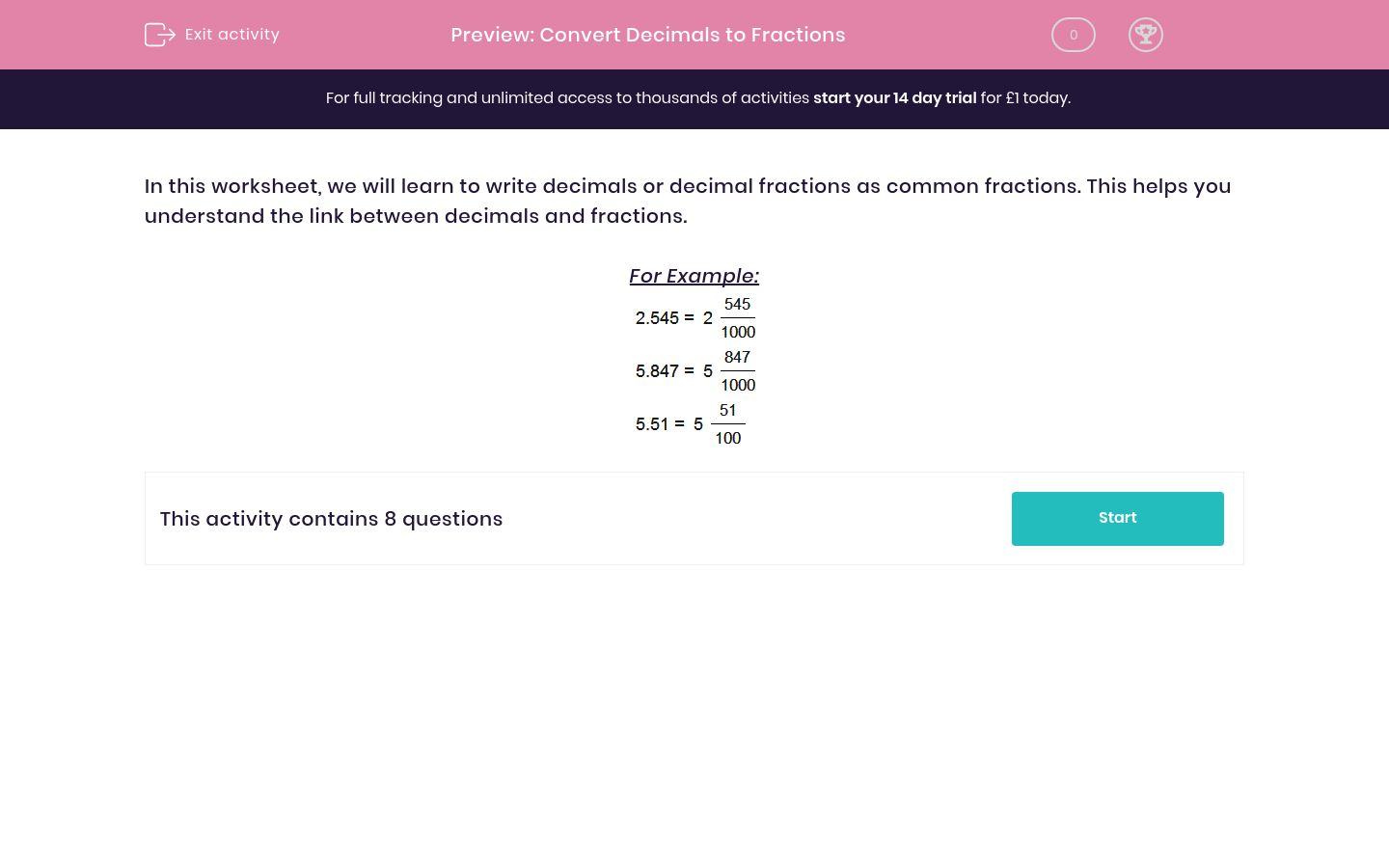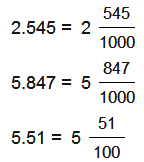# Convert Decimals to Fractions

In this worksheet, students practise changing decimal numbers to their fraction equivalents.Key stage:  KS 2

Curriculum topic:   Maths and Numerical Reasoning

Curriculum subtopic:   Decimals

Difficulty level:### QUESTION 1 of 10

In this worksheet, we will learn to write decimals or decimal fractions as common fractions. This helps you understand the link between decimals and fractions.

For Example:Write the decimal as a common fraction by filling in the gap.

2.607
=
2
 1000

Write the decimal as a common fraction by filling in the gap.

0.295
=

 1000

Write the decimal as a common fraction by filling in the gap.

8.097
=
8
 1000

Write the decimal as a common fraction by filling in the gap.

5.29
=
5
 100

Write the decimal as a common fraction by filling in the gap.

5.864
=
5
 1000

Write the decimal as a common fraction by filling in the gap.

2.293
=
2
 1000

Write the decimal as a common fraction by filling in the gap.

3.69
=
3
 100

Write the decimal as a common fraction by filling in the gap.

5.002
=
5
 1000

• Question 1

Write the decimal as a common fraction by filling in the gap.

2.607
=
2
 1000

607
• Question 2

Write the decimal as a common fraction by filling in the gap.

0.295
=

 1000

295
• Question 3

Write the decimal as a common fraction by filling in the gap.

8.097
=
8
 1000

97
• Question 4

Write the decimal as a common fraction by filling in the gap.

5.29
=
5
 100

29
• Question 5

Write the decimal as a common fraction by filling in the gap.

5.864
=
5
 1000

864
• Question 6

Write the decimal as a common fraction by filling in the gap.

2.293
=
2
 1000

293
• Question 7

Write the decimal as a common fraction by filling in the gap.

3.69
=
3
 100

69
• Question 8

Write the decimal as a common fraction by filling in the gap.

5.002
=
5
 1000

2
---- OR ----

Sign up for a £1 trial so you can track and measure your child's progress on this activity.

### What is EdPlace?

We're your National Curriculum aligned online education content provider helping each child succeed in English, maths and science from year 1 to GCSE. With an EdPlace account you’ll be able to track and measure progress, helping each child achieve their best. We build confidence and attainment by personalising each child’s learning at a level that suits them.

Get started